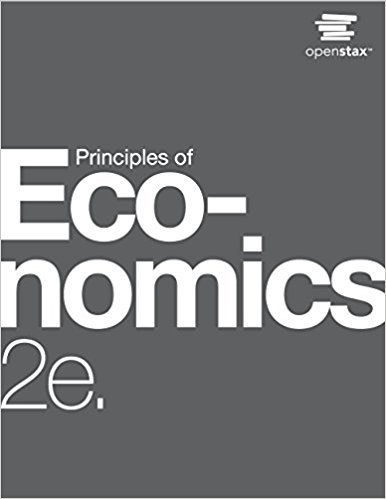×
Get Full Access to Principles Of Economics - 2 Edition - Chapter 2 - Problem 5
Get Full Access to Principles Of Economics - 2 Edition - Chapter 2 - Problem 5

×

# Solved: Individuals may not act in the rational, calculating way described by theISBN: 9781947172364 471

## Solution for problem 5 Chapter 2

Principles of Economics | 2nd Edition

• Textbook Solutions
• 2901 Step-by-step solutions solved by professors and subject experts
• Get 24/7 help from StudySoup virtual teaching assistantsPrinciples of Economics | 2nd Edition

4 5 1 378 Reviews
11
2
Problem 5

Individuals may not act in the rational, calculating way described by the economic model of decision making, measuring utility and costs at the margin, but can you make a case that they behave approximately that way?

Step-by-Step Solution:
Step 1 of 3

Step 2 of 3

Step 3 of 3

##### ISBN: 9781947172364

This full solution covers the following key subjects: . This expansive textbook survival guide covers 37 chapters, and 1291 solutions. The full step-by-step solution to problem: 5 from chapter: 2 was answered by , our top Business solution expert on 03/16/18, 04:24PM. Principles of Economics was written by and is associated to the ISBN: 9781947172364. Since the solution to 5 from 2 chapter was answered, more than 318 students have viewed the full step-by-step answer. This textbook survival guide was created for the textbook: Principles of Economics, edition: 2. The answer to “Individuals may not act in the rational, calculating way described by the economic model of decision making, measuring utility and costs at the margin, but can you make a case that they behave approximately that way?” is broken down into a number of easy to follow steps, and 36 words.

Unlock Textbook Solution